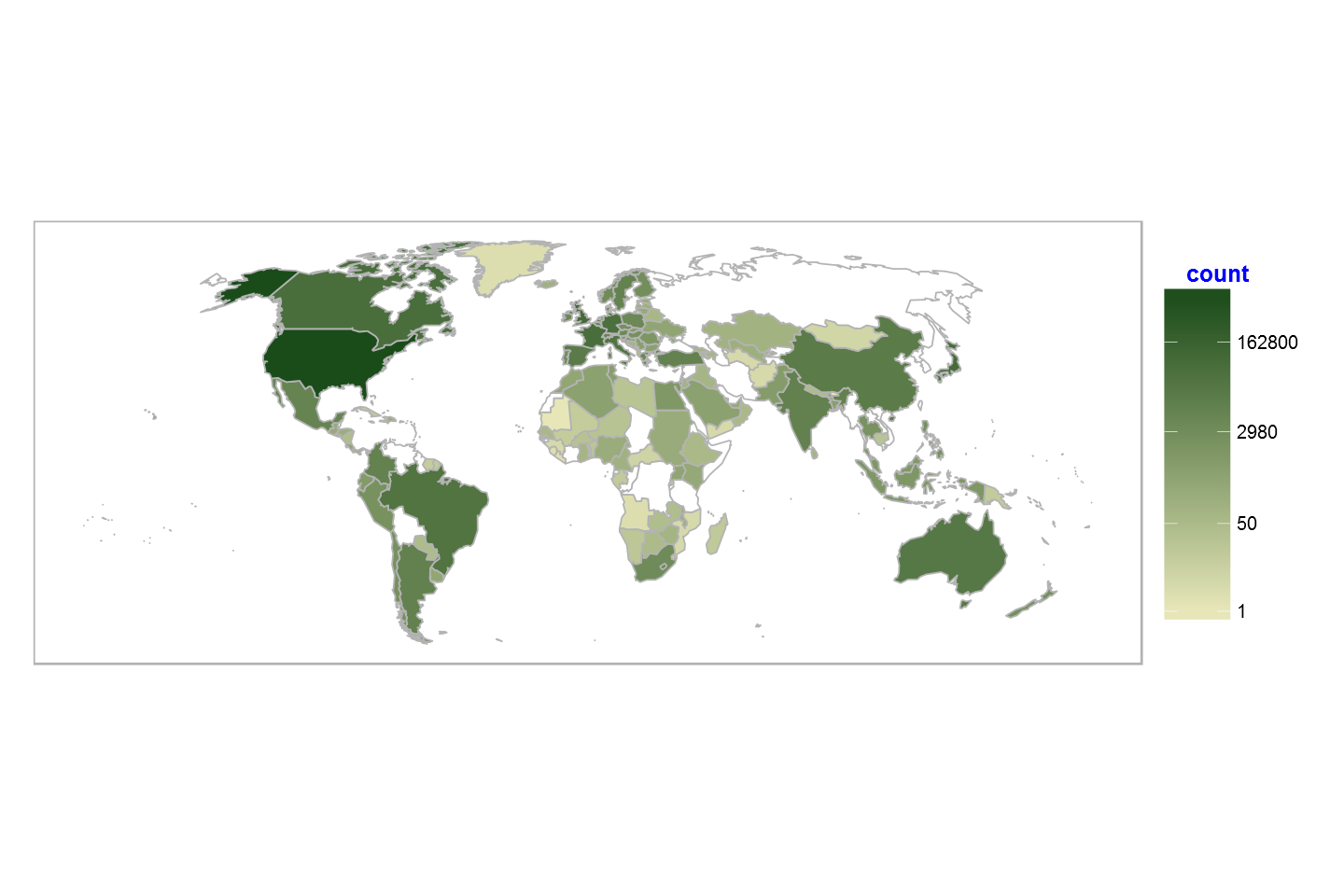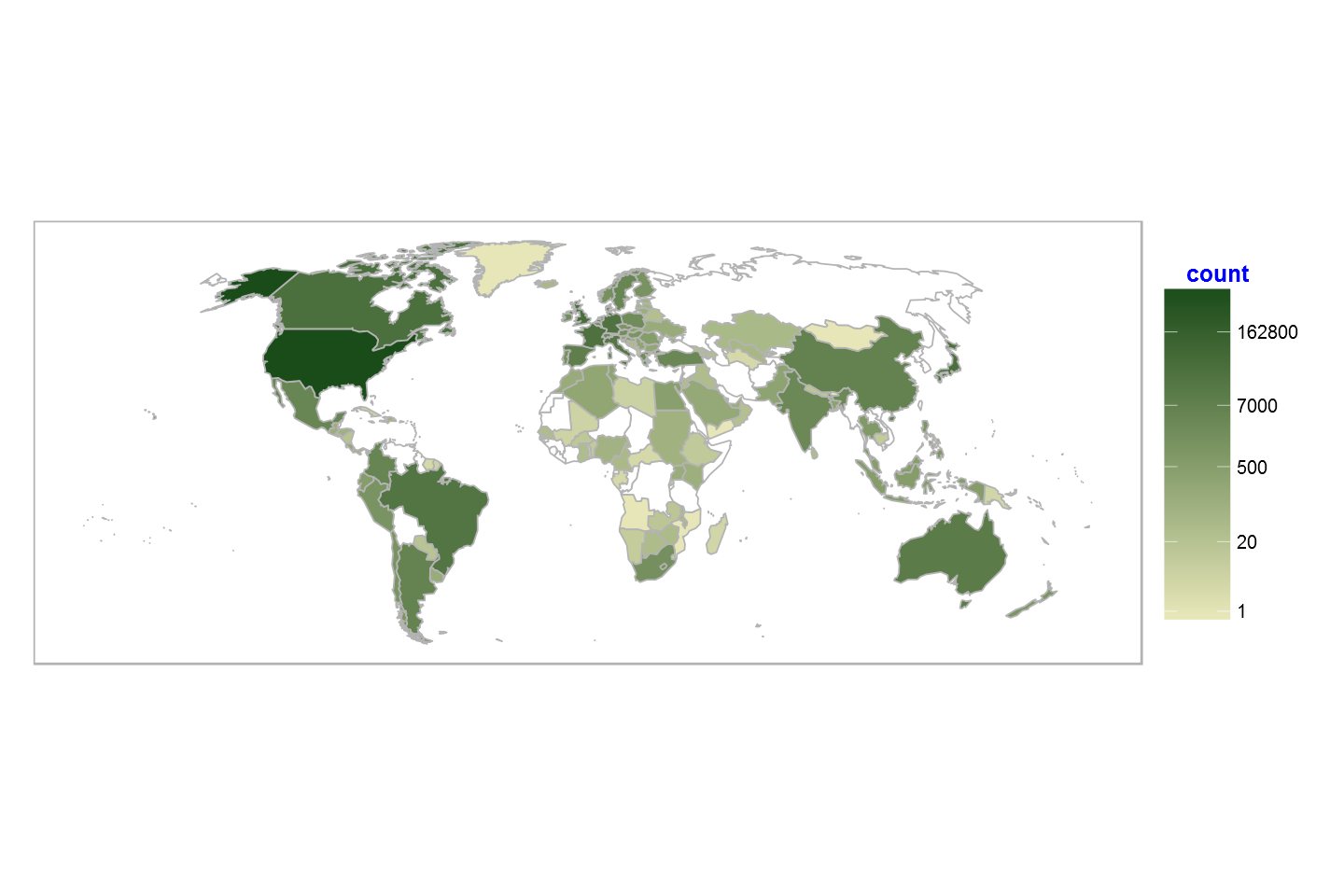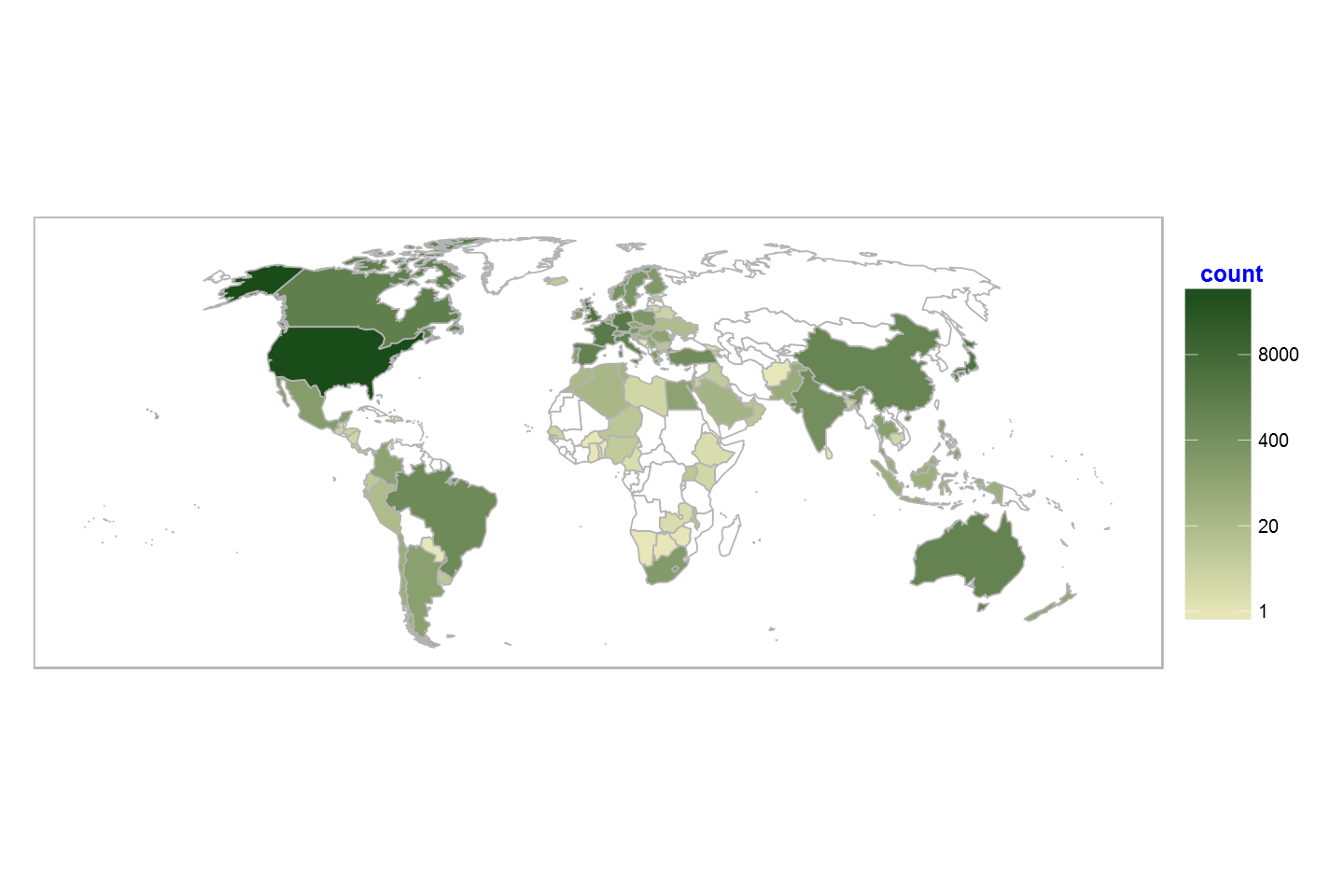# Fisseha Berhane, PhD

#### Data Scientist443-970-2353[email protected] CV Resume### Spatial distribution of FDA's drug adverse events report¶

"The FDA Adverse Event Reporting System (FAERS) is a database that contains information on adverse event and medication error reports submitted to FDA."

The FAERS data are at quarterly intervals and they contain the following:

Demographic and administrative information and the initial report image ID number (if available);

Drug information from the case reports;

Reaction information from the reports;

Patient outcome information from the reports;

Information on the source of the reports;

A "README" file containing a description of the files.

In this post, let's see the spatial distribution of the FAERS data. We will download the quarterly data from this. Then, concatenate the quartely data to create one dataset. The data covers since the fourth quarter of 2012 to the second quarter of 2015 (at the time of writing this post).

In :
require(downloader)
library(dplyr)

In :
year_start=2012
year_last=as.integer(format(Sys.Date(), "%Y"))

for (i in year_start:year_last){

if (i==2012){
url<-"http://www.nber.org/fda/faers/2012/demo2012q4.csv.zip"

unzip ("data.zip")
}

else{
if (i<year_last){
j=c(1:4)

for (m in j){

url<-paste0("http://www.nber.org/fda/faers/",i,"/demo",i,"q",m,".csv.zip")

unzip ("data.zip")
}
}

else if (i==year_last){
j=c(1:2)
for (m in j){

url<-paste0("http://www.nber.org/fda/faers/",i,"/demo",i,"q",m,".csv.zip")

unzip ("data.zip")

}
}

}

}


After downloading the quartely data sets, let's concatenate them. The unzipped data set for the first quarter of 2013 is demo2013q1.csv

The demographic data contains various variables. Let's select "primaryid", "occr_country" and "sex". "occr_country" is the country where the adverse event took place.

We can use the select function from the dplyr package.

In :
demo = select(read.csv("demo2012q4.csv",stringsAsFactors=F),primaryid,occr_country,sex)

for (i in year_start+1:year_last){

if (i<year_last){
j=c(1:4)

for (m in j){

}
}

else if (i==year_last){
j = c(1:2)

for (m in j){
}
}

}


The "occr_country" variable in the FAERS data is two letter country code. We can map the code to the country name using the appropriate codes from this website.

In :
library(ggplot2)
library(jsonlite)
library(lubridate)
library(grid)     # for 'unit'
library(scales)   # for 'percent'
library(rgdal)    # working with shapefile
library(dplyr)    # awesome data manipulation
library(tidyr)    # reshaping data
library(ggthemes) # theme_map
library(gridExtra)


Let's change the variable name "occr_country" to "Code" to merge it with the country names and codes data.

In :
demo2=rename(demo,Code=occr_country)

In :
c_codes<-read.csv("http://data.okfn.org/data/core/country-list/r/data.csv")


Now, let's merge the demography (demo2) data with the c_codes, which contains country codes and full names.

In :
demo2=merge(demo2, c_codes,by ="Code",all.x = TRUE) #left outer join

demo2$id=demo2$Name   # we will use this variable later.


In :
try(invisible(GET("http://www.pewglobal.org/wp-content/lib/js/world-geo.json",
write_disk("world-geo.json"))), silent=TRUE)

In :
world <- readOGR("world-geo.json", "OGRGeoJSON")
world_wt <- spTransform(world, CRS("+proj=robin"))
worldmap <- fortify(world_wt)

worldmap %>%
left_join(data_frame(id=rownames(world@data), name=world@data$name)) %>% select(-id) %>% rename(id=name) -> worldmap  OGR data source with driver: GeoJSON Source: "world-geo.json", layer: "OGRGeoJSON" with 243 features and 1 fields Feature type: wkbMultiPolygon with 2 dimensions  Regions defined for each Polygons Joining by: "id"  Let's calculate adverse event reports by country and merge it with the geography data. In : count_all<-demo2%>%group_by(id)%>%summarise(count = n()) world_map = merge(worldmap, count_all,by ="id",all.x = TRUE) # Reorder the data world_map = world_map[order(world_map$group, world_map$order),]  Now, let's calculate adverse event reports by county and sex (female, male and unknown or not reported) In : count_gender<-demo2%>%group_by(id,sex)%>%summarise(count = n()) world_map_gender = merge(worldmap, count_gender,by ="id",all.x = TRUE) # Reorder the data world_map_gender = world_map_gender[order(world_map_gender$group, world_map_gender$order),] world_map_M=world_map_gender[world_map_gender$sex=="M",]  # male
world_map_F=world_map_gender[world_map_gender$sex=="F",] # Female world_map_NSUNK=world_map_gender[world_map_gender$sex%in%c("UNK","NS"),] #unknown or not specified

world_map_M2 = merge(worldmap, world_map_M,by ="id",all.x = TRUE)
world_map_M3 =world_map_M2[order(world_map_M2$group.x, world_map_M2$order.x),]

world_map_F2 = merge(worldmap, world_map_F,by ="id",all.x = TRUE)
world_map_F3 =world_map_F2[order(world_map_F2$group.x, world_map_F2$order.x),]

world_map_NSUNK2 = merge(worldmap, world_map_NSUNK,by ="id",all.x = TRUE)
world_map_NSUNK3 =world_map_NSUNK2[order(world_map_NSUNK2$group.x, world_map_NSUNK2$order.x),]


Now, using ggplot2, let's produce maps of adverse events by country (for all genders) and for each gender separately. Let's use log transformation.

In :
f_breaks = c(1, 20, 500, 7000,162800)

female<-ggplot(world_map_F3, aes(x=long.x, y=lat.x, group=group.x)) +
geom_polygon(aes(fill=count), color="gray70") +
scale_fill_gradient(name = "count",breaks = f_breaks, labels = f_breaks, trans = "log",
low = "#E6E6B8", high = "#1A4C1A",guide = "colorbar",na.value="white")+
theme(axis.text.y   = element_blank(),
line = element_blank(),
axis.text.x   = element_blank(),
axis.title.y  = element_blank(),
axis.title.x  = element_blank(),
panel.background = element_blank(),
panel.grid.major = element_blank(),
panel.grid.minor = element_blank(),
panel.border = element_rect(colour = "gray70", fill=NA, size=1))+
theme(aspect.ratio=0.4,legend.key.size = unit(1.5, "cm"),
legend.title = element_text(size = 15, colour = "blue"),
legend.title.align=0.3,legend.text = element_text(size = 12))

In :
m_breaks = c(1, 20, 600, 9000,162800)

male<-ggplot(world_map_M3, aes(x=long.x, y=lat.x, group=group.x)) +
geom_polygon(aes(fill=count), color="gray70") +
scale_fill_gradient(name = "count",breaks = m_breaks, labels = m_breaks, trans = "log",
low = "#E6E6B8", high = "#1A4C1A",guide = "colorbar",na.value="white")+

theme(axis.text.y   = element_blank(),
line = element_blank(),
axis.text.x   = element_blank(),
axis.title.y  = element_blank(),
axis.title.x  = element_blank(),
panel.background = element_blank(),
panel.grid.major = element_blank(),
panel.grid.minor = element_blank(),
panel.border = element_rect(colour = "gray70", fill=NA, size=1))+

theme(aspect.ratio=0.4,legend.key.size = unit(1.5, "cm"),legend.title = element_text(size = 15, colour = "blue"),
legend.title.align=0.3,legend.text = element_text(size = 12))

In :
u_breaks = c(1, 20, 400, 8000)

unknown_sex<-ggplot(world_map_NSUNK3, aes(x=long.x, y=lat.x, group=group.x)) +
geom_polygon(aes(fill=count), color="gray70") +
scale_fill_gradient(name = "count", trans = "log",breaks = u_breaks, labels = u_breaks,
low = "#E6E6B8", high = "#1A4C1A",guide = "colorbar",na.value="white")+

theme(axis.text.y   = element_blank(),
line = element_blank(),
axis.text.x   = element_blank(),
axis.title.y  = element_blank(),
axis.title.x  = element_blank(),
panel.background = element_blank(),
panel.grid.major = element_blank(),
panel.grid.minor = element_blank(),
panel.border = element_rect(colour = "gray70", fill=NA, size=1))+
theme(aspect.ratio=0.4,legend.key.size = unit(1.5, "cm"),
legend.title = element_text(size = 15, colour = "blue"),
legend.title.align=0.3,legend.text = element_text(size = 12))

In :
all_breaks = c(1, 50, 2980, 162800)

all<-ggplot(world_map, aes(x=long, y=lat, group=group)) +
geom_polygon(aes(fill=count), color="gray70") +

scale_fill_gradient(name = "count", trans = "log",breaks = all_breaks, labels = all_breaks,
low = "#E6E6B8", high = "#1A4C1A",guide = "colorbar",na.value="white")+

theme(axis.text.y   = element_blank(),
line = element_blank(),
axis.text.x   = element_blank(),
axis.title.y  = element_blank(),
axis.title.x  = element_blank(),
panel.background = element_blank(),
panel.grid.major = element_blank(),
panel.grid.minor = element_blank(),
panel.border = element_rect(colour = "gray70", fill=NA, size=1))+theme(aspect.ratio=0.4,legend.key.size = unit(1.5, "cm"),

legend.title = element_text(size = 15, colour = "blue"),
legend.title.align=0.3,legend.text = element_text(size = 12))


Now, we can simply call the map objects.

#### Adverse event reports by country:¶

In :
all

Out:#### Adverse event reports by country for females:¶

In :
female

Out:#### And finally, unknown or not specified gender:¶

In :
unknown_sex

Out: